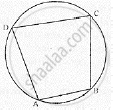Share

Books Shortlist

# In Cyclic Quadrilateral Abcd, ∠A = 3 ∠C and ∠D = 5 ∠B. Find the Measure of Each Angle of the Quadrilateral. - ICSE Class 10 - Mathematics

#### Question

In cyclic quadrilateral ABCD, ∠A = 3 ∠C and ∠D = 5 ∠B. Find the measure of each angle of the quadrilateral.

#### Solution∴ ∠A + ∠C  = 180°
⇒3∠C + ∠C = 180°

⇒ 4∠C  = 180°
⇒ ∠C = 45°
∵ ∠A  = 3∠C
⇒ ∠A = 3 × 45°
⇒ ∠A = 135°
Similarly,
∴ ∠B + ∠D  = 180°
⇒ ∠B + 5∠B  = 180°

⇒ 6∠B  = 180°
⇒ ∠B = 30°
∵ ∠D =  5∠B
⇒ ∠D =  5 × 30°
⇒ ∠D  = 150°
Hence, ∠A  = 135°  ,∠B = 30°  , ∠C = 45°  ,∠D  =150°

Is there an error in this question or solution?

#### Video TutorialsVIEW ALL 

Solution In Cyclic Quadrilateral Abcd, ∠A = 3 ∠C and ∠D = 5 ∠B. Find the Measure of Each Angle of the Quadrilateral. Concept: Cyclic Properties.
S